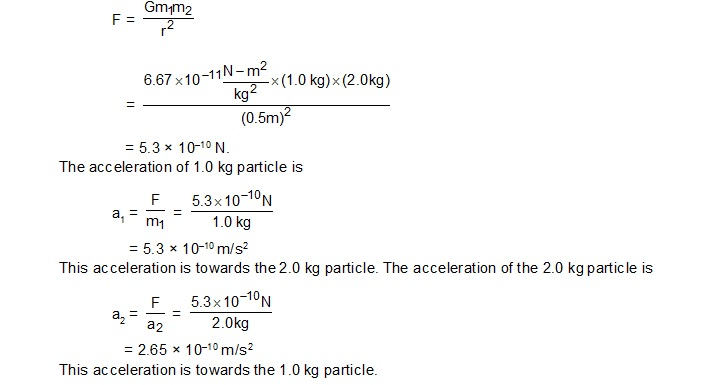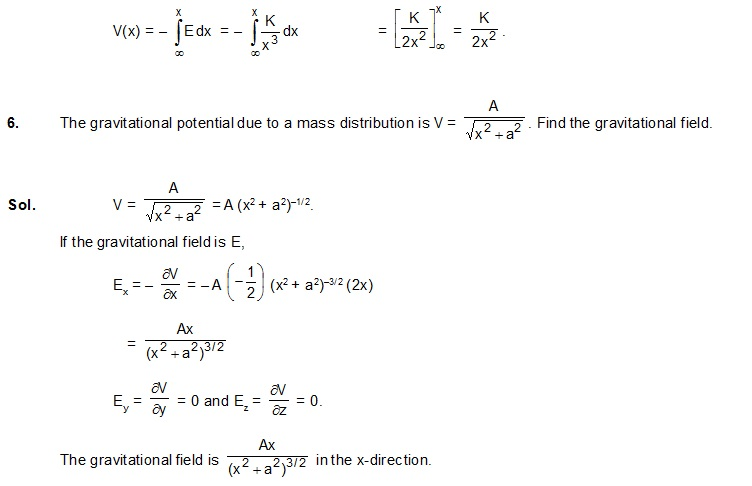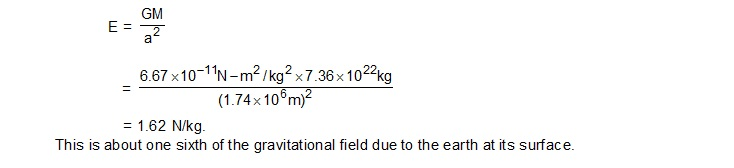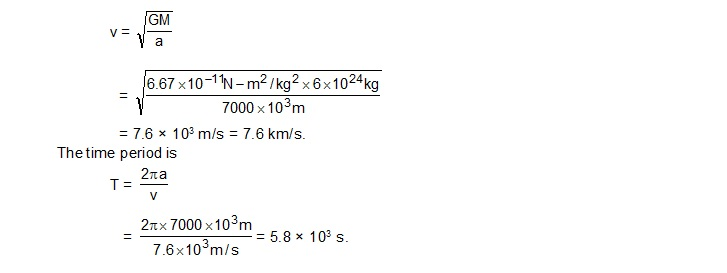# Solved Examples

1.    Two particles of masses 1.0 kg and 2.0 kg are placed at a separation of 50 cm. Assuming that the only forces acting on the particles are their mutual gravitation, find the initial accelerations of the two particles.
Sol.    The force of gravitation exerted by one particle on another is2.    Find the work done in bringing three particles, each having a mass of 100 g, from large distances to the vertices of an equilateral triangle of side 20 cm.

Sol.    When the separation are large, the gravitational potential energy is zero. When the particles are brought at the vertices of the triangle ABC, three pairs AB, BC and CA are formed. The potential energy of each pair is – Gm1m2/r and hence the total potential energy becomes= – 1.0 × 10–11 J.
The work done by the gravitational forces is W = – U = 1.0 × 10–11 J. If the particles are brought by some external agency without changing the kinetic energy, the work done by the external agency is equal to the change in potential energy = – 1.0 × 10–11 J.

3.    A particle of mass M is placed at the centre of a uniform spherical shell of equal mass and radius a. Find the gravitational potential at a point P at a distance a/2 from the centre.

Sol.    The gravitational potential at the point P due to the particle at the centre is
V1 = –GM/(a/2)  = –2GM/a .
The potential at P due to the shell is
V2 = –GM/a
The net potential at P is V1 + V2 = – .3GM/a

4.    A particle of mass 50 g experiences a gravitational force of 2.0 N when placed at a particular point. Find the gravitational field at that point.

Sol.    The gravitational field has a magnitude5.    The gravitational field due to a mass distribution is given by E = K/x3 in X-direction. Taking the gravitational potential to be zero at infinity, find its value at a distance x.      HCV_Ch-11_Sol.Ex._5
Sol.The potential at a distance x is7.    Find the gravitational field due to the moon at its surface. The mass of the

moon is 7.36 × 1022 kg and the radius of the moon is 1.74 × 106 m. Assume the moon to be a spherically symmetric body.

Sol.    To calculate the gravitational field at an a external point, the moon may be replaced by a single particle of equal mass placed at its centre. Then the field at the surface is8.    Calculate the value of acceleration due to gravity at a point (a) 5.0 km above the earth’s surface and (b) 5.0 km below the earth’s surface. Radius of earth = 6400 km and the value of g at the surface of the earth is 9.80 m/s2.9.    A satellite is revolving round the earth at a height of 600 km. Find (a) the speed of the satellite and (b) the time period of the satellite. Radius of the earth = 6400 km and mass of the earth = 6 × 1024 kg.

Sol.    The distance of the satellite from the centre of the earth is 6400 km + 600 km = 7000 km.
The speed of the satellite is10.    Calculate

• 1
• 2
• 3
• 4
• 5
• 6
• 7
• 8
• 9
• 10
• 11